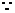2.9 HCF and LCM of Polynomials/Algebraic expressions:

We use the division method to find HCF and LCM of monomials or binomials or trinomials or any algebraic expressions

2.9 Problem 1: Find HCF and LCM of  (p+3)3, 2p3+54+18p(p+3), (p2+6p+9)

Solution:

Step 1: Find factors of all the expressions first.

1.The factors of (p+3)3 are (p+3),(p+3) and (p+3)

2. Let us simplify the 2nd term:

2p3+54+18p(p+3)

= 2(p3+27)+18p(p+3)

= 2*(p+3)( p2+9-3p)+18p(p+3),  (p3+27 is of the form a3+b3 with a=p and b=3we can apply the formula a3+b3 =(a+b) (a2 +b2 -ab)

=(p+3)*((2*(p2+9-3p))+18p)

= (p+3) *2*( p2+9-3p+9p)

=2(p+3)( p2+9+6p) ((p2+9+6p) is of the form ( a2+ b2+2ab) with a=p and b=3, but ( a2+ b2+2ab)= (a+b)2

= 2(p+3)(p+3)2The factors of 2p3+54+18p(p+3) are 2, (p+3),(p+3),(p+3)

3. we have already seen above that (p2+6p+9) =(p+3)2The factors of (p2+6p+9) are p+3, p+3

Follow the division method to find HCF and LCM

The given terms can be rewritten as ( p+3)(p+3)(p+3),  2(p+3)(p+3)(p+3), (p+3)(p+3)

The first common factor is p+3 and let us start dividing terms by this term

(p+3) | ( p+3)(p+3)(p+3),  2(p+3)(p+3)(p+3), (p+3)(p+3)

(p+3) | (p+3)(p+3),            2(p+3)(p+3),          (p+3)

(p+3),                     2(p+3)                            1

We stop further division as there are no more common factors for all the terms

Therefore HCF = (p+3)(p+3)= (p+3)2  and

(p+3) | ( p+3)(p+3)(p+3),  2(p+3)(p+3)(p+3), (p+3)(p+3)

(p+3) | (p+3)(p+3),            2(p+3)(p+3),          (p+3)

(p+3) | (p+3),                     2(p+3)                            1

1,                          2,                                    1

We stop further division as there are no more common factors for any 2 terms

Therefore LCM = (p+3)(p+3)(p+3)*1*2*1 = 2(p+3)3

Verification:

Let us cross check the solution by substituting p=2 in the above problem.

From the solution the HCF is (p+3)2 = (2+3)2 =25 and LCM= 2(p+3)3= 2(2+3)3= 2*125=250

Let us find the HCF and LCM of the given terms after converting them to numbers by substituting p=2.

Therefore the terms are  (2+3)3, (2*23+54+18*2(2+3)), (22+6*2+9)

= {125, 250,25}

By close observation we notice that HCF=25 and LCM=250

Since both the methods give same HCF and LCM our solution is correct.

2.9 Problem 2: Find HCF and LCM of  10(x2-y2), 15(x2-2xy+y2) 20(x3- y3),5(-3x +3y)

Solution:

Step 1: Find factors of all the expressions first.

1. The first term has an expression of the form  (a2-b2) whose factors are (a+b) and (a-b) with a=x and b= yThe factors of first term are 10, (x+y) and (x-y)10(x2-y2)=10(x+y)(x-y)

2. The second term has an expression of the form (a2-2ab+b2) whose factors are (a-b) and (a-b) with a=x and b= yThe factors of second term are 15, (x-y) and (x-y)15(x2-2xy+y2)= 15(x-y) (x-y)

3. The third term has an expression of the form (x3-y3) whose factors are (x-y) and (x2 +y2 +xy) with a=x and b=yThe factors of third term are  20, (x-y) and (x2 +y2 +xy)

4. The fourth term can be rewritten as 5*-3(x-y)The factors of fourth term are -15, (x-y)5*-3(x-y) = 5*(-3)(x-y)=-15, (x-y)

Step 2: Follow the division method to find HCF and LCM

The common factors are 5 and (x-y) so let us start dividing terms by these two together

5 (x-y) | 10(x+y) (x-y), 15(x-y) (x-y), 20(x-y)(x2 +y2 +xy), -15(x-y)

2(x+y),             3(x-y),             4(x2 +y2 +xy),        -3

We stop further division as there are no more common factors for all the terms

Therefore HCF = 5(x-y)

To find LCM, we start division with 5(x-y)

5(x-y) | 10(x+y) (x-y), 15(x-y) (x-y), 20(x-y)(x2 +y2 +xy), -15(x-y)

2|  2(x+y),             3(x-y),             4(x2 +y2 +xy),        -3 (We continue division as some terms have common factors)

3|   (x+y),             3(x-y),              2(x2 +y2 +xy),        -3

(x+y),                (x-y),              2(x2 +y2 +xy)         -1

We stop further division as there are no common factors among any 2 terms

Therefore LCM =5(x-y)* 2*3*(x+y)*(x-y)*2(x2 +y2 +xy)

=  60*(x-y)(x+y)*(x-y)(x2 +y2 +xy) (Note that (x-y)(x2 +y2 +xy) is of the form (a-b)( (a2 +b2 +ab)   with a=x and b= y)

= 60*(x2-y2)* (x3-y3)

Verification:

Since it is very difficult to cross verify easily, we will cross check the solution for at least  for one value of x and y by substituting x=3 and y=2 in the above problem

From the solution the HCF is 5(x-y) = 5*(3-2) = 5  and

LCM= 60*(x2- y2)* (x3-y3) = 60*(9-4)*)(27-8)=60*5*19=5700

Let us find the HCF and LCM of the given terms after converting them to numbers by substituting x=3 and y=2 in

10(x2-y2), 15(x2-2xy+y2) 20(x3- y3),5(-3x +3y)

Therefore the terms are 10(32-22), 15(32-2*3*2+22), 20(33- 23),5(-3*3 +3*2)

= {50, 15, 380, -15}

By observation we note that HCF=5

Let us use the division method to find LCM

5 | 50,15,380,-15

2 | 10,3,76,-3

3 | 5,3,38,-3

|  5,1,38,-1

LCM = 5*2*3*5*38=5700

Since both the methods give same HCF and LCM our solution is not incorrect.

2.9 Problem 3 : For what value of  a and b the polynomials

p(x) = (x2+3x+2) (x2+2x+a) and

q(x) = (x2+7x+12) (x2+7x+b)

have (x+1)(x+3) as their HCF

Solution:

(x2+3x+2) = (x+1)(x+2)

(x2+7x+12) = (x+4)(x+3)p(x) = (x+1)(x+2)(x2+2x+a)

q(x) = (x+4)(x+3) (x2+7x+b)

Since it is given that (x+1)(x+3) is HCF of  p(x),we conclude that

(x+3) is factor of (x2+2x+a)

This implies x=-3 satisfies the equation (x2+2x+a) =0(-3)2+2(-3)+a =0

I.e. 9-6+a =0a =-3

Since it is given that (x+1)(x+3) is HCF of  q(x),we conclude that

(x+1) is factor of (x2+7x+b)

This implies x=-1 satisfies the equation (x2+7x+b) =0(-1)2+7(-1)+b =0

I.e. 1-7+b =0b =6

Verfication :  By substituting value for a an b in p(x) and q(x) we get

p(x) = (x2+3x+2) (x2+2x-3) = (x+1) (x+2) (x+3) (x-1) {(x2+2x-3) = (x+3)(x-1)}

q(x) =(x2+7x+12) (x2+7x+6) = (x+4) (x+3) (x+1) (x+6) {(x2+7x+6)= (x+1)(x+6)}

By looking at factors of p(x) and q(x) we conclude that HCF of p(x) and q(x) is (x+1) (x+3)

2.9 Summary of learning

 No Points studied 1 Division method to find HCF and LCM of algebraic expressions